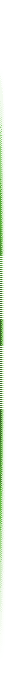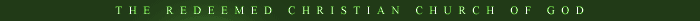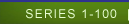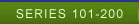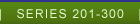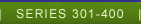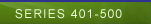SERIES 301-400
 LESSON 301- 325 Select Lesson Lesson 301 Lesson 302 Lesson 303 Lesson 304 Lesson 305 Lesson 306 Lesson 307 Lesson 308 Lesson 309 Lesson 310 Lesson 311 Lesson 312 Lesson 313 Lesson 314 Lesson 315 Lesson 316 Lesson 317 Lesson 318 Lesson 319 Lesson 320 Lesson 321 Lesson 322 Lesson 323 Lesson 324 Lesson 325 LESSON 326- 351 Select Lesson Lesson 326 Lesson 327 Lesson 328 Lesson 329 Lesson 330 Lesson 331 Lesson 332 Lesson 333 Lesson 334 Lesson 335 Lesson 336 Lesson 337 Lesson 338 Lesson 339 Lesson 340 Lesson 341 Lesson 342 Lesson 343 Lesson 344 Lesson 345 Lesson 346 Lesson 347 Lesson 348 Lesson 349 Lesson 350 Lesson 351 LESSON 351-378 Select Lesson Lesson 351 Lesson 352 Lesson 353 Lesson 354 Lesson 355 Lesson 356 Lesson 357 Lesson 358 Lesson 359 Lesson 360 Lesson 361 Lesson 362 Lesson 363 Lesson 364 Lesson 365 Lesson 366 Lesson 367 Lesson 368 Lesson 369 Lesson 370 Lesson 371 Lesson 372 Lesson 373 Lesson 374 Lesson 375 Lesson 376 Lesson 377 Lesson 378 LESSON 379-398 Select Lesson Lesson 379 Lesson 380 Lesson 381 Lesson 382 Lesson 383 Lesson 384 Lesson 385 Lesson 386 Lesson 387 Lesson 388 Lesson 389 Lesson 390 Lesson 391 Lesson 392 Lesson 393 Lesson 394 Lesson 395 Lesson 396 Lesson 397 Lesson 398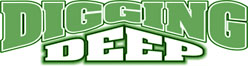LESSON 301 ELISHA A STUDY IN DOUBLE ANOINTING - PART 9 INTRODUCTION: In the last part of this series, we saw how anointing can be carried by words of the anointed to remove the “buts” in any life or to inflict punishment on sinners. We shall now continue our study by examine two different life situations in II Kings 6. May the Lord bless us richly as we study in Jesus Name. Amen. 1. ANOINTING IS THE GREATEST INSURANCE AGAINST DISASTER Anointing has its' own problems, but they are good problems Luke.1:37; II Kings 6:1-7. Those who solve the problems of the anointed are solving their own problems too I Kings 17:8-16; II Kings 4:8-17. Moreover, those who know the value of anointing never stay afar from the anointed because anointing is the greatest insurance against disaster Ps. 34:5; II Kings 2:2-6. 2. THE ANOINTING HAS AN UNSEEN BODYGUARD The anointed is endowed with supernatural audio-visual powers, which frustrate the plans of the enemy II Kings 6:8-13; II Kings 5:26. This is one of the reasons why the devil respects the anointed Judges 15:11-16; Heb. 13:5-6. Moreover, anointing can make the blind see and the seeing blind II Kings 1:9-14; John 9:40-41; Acts 13:8-12; also the anointing has an unseen body guard John 1:45-50; John 14:16. Further Readings: Matt. 8:41-45; I Sam. 22:1-2.